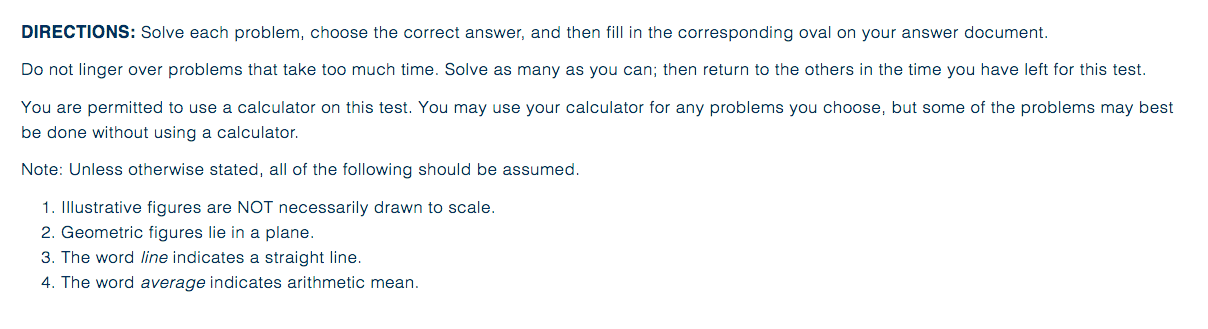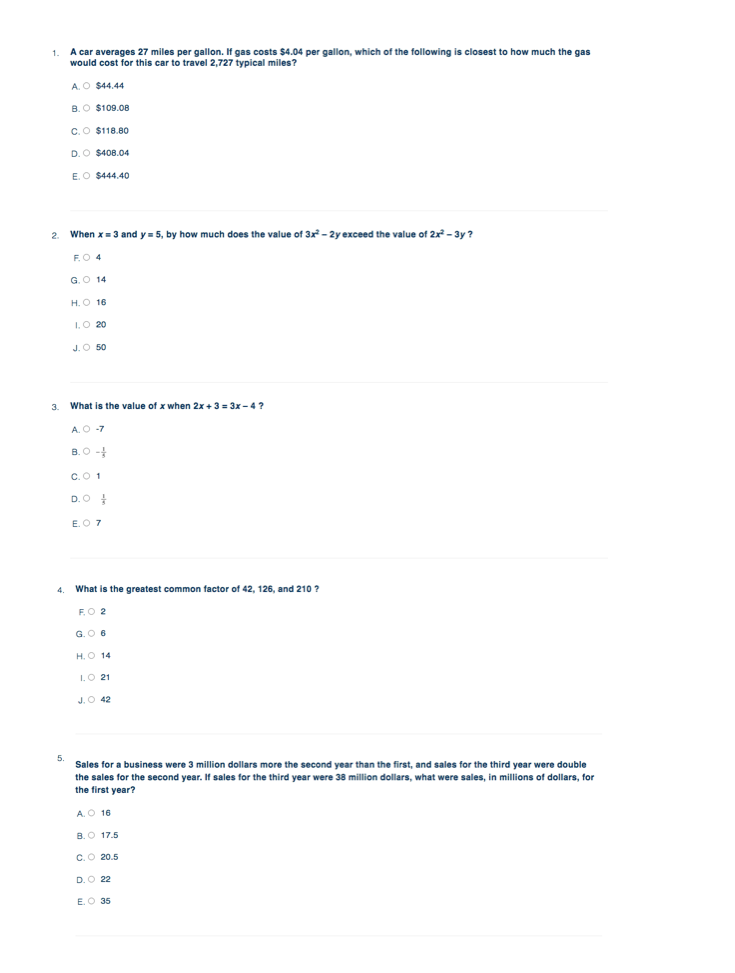# ACT Math Practice Test

Guide to ACT Math Practice Tests & Answering Confusing ACT Questions

##Understand ACT Practice Math Tests & Tips to Improve Your ACT Score

Taking Math ACT practice tests is the best way to study for the ACT test and improve your composite score. The ACT Math Test is a standardized test that tests your mathematical reasoning ability to understand basic math terminology and recall math principles and formulas. It is a 60 questions section that you must complete within 60 minutes.

The test will evaluate the test taker’s math skills acquired through grade 12. The test is available in multiple-choice questions, which require reasoning skills to solve. The ACT Math practice test is a test you can take as you prepare for the final test, and it is in the same format and style with the same questions you expect to find in the final exam.

The test is time conscious, and the practice test will help you prepare in time management and hone skills where you are weak. Let’s review some ACT Practice Tests for Math and analyze some tips & tricks to help answer confusing test questions.

## ACT Math Practice Test

Here is an ACT Math Practice Test originally published by the ACT for students to prepare for the ACT Test and test their mathematics skills covered on the ACT math section.### ACT Math Practice QuestionsThis practice test along with its questions, answers, and explanations is provided by the ACT and is it’s copyright.

## Should You Take ACT Math Practice Tests?

The ACT Math practice test, like most practice tests, is essential for everyone at any stage of preparation, from those just beginning to studying to those who have been studying for months. Surprisingly, the practice test’s capabilities are limited.

The practice test helps you study in various methods, enabling you to prepare for the real test. Taking the test will, in particular, assist you in preparing for the ACT Math test.

It’s vital to remember that the practice test is really beneficial while taking them since not only do you learn a lot, but it also allows you to reflect afterward. After taking practice tests and analyzing your performance, you will discover when it is more productive to take the test.

## How many ACT Math Practice Questions Should I Take?

You should take as many practice tests as you can. Anticipating the ACT Math test is nerve-wracking, and it can be challenging to figure out how you should prepare.

Practices tests are a good way of preparing for the ACT since they give you a view of what the test will look like so that you are not surprised on the final test day. But the question is, how many practice tests are recommended?

According to experts, you should take three to five practice tests before sitting for the final test. But it is vital to note that taking the tests is no assurance that your scores will improve, and you should evaluate your performance after each practice test. Evaluating performance will help you in identifying weak areas where you can improve.

## ACT Math Section Question Types, Format, & Topics Covered

ACT Math section comprises 60 questions that should be attempted in 60 minutes. The questions require the test taker to use reasoning abilities to attempt the questions. The questions will not be listed according to topics, but most topics covered geometry, statistics, and algebra. ACT math problems will be straightforward for advanced students

There are six question types, and Algebra will have 14 questions, ten elementary algebra questions, nine intermediary algebra questions. Geometry comprises 23 questions, with 14 in plane geometry and nine on coordinate geometry. Four questions will be from trigonometry.

## How to get the Most Out of Math ACT Practice Tests

One tip for the ACT test that’s always relevant no matter what section you are taking is time management. When you sit down to take the practice test, you should make the most of your time. Because you will be taking the ACT on paper, the first act is to print the test and practice on paper. You should keep track of your time since you have 60 minutes.

You will need at most one minute per question to complete the 60 questions. After you’ve finished the practice test, go through your answers for all problems to see where you may make improvements.

Take between three and five tests to become familiar with the test and, in some situations where you find difficulties, seek assistance to identify your deficiencies and areas for improvement.

## ACT Math Practice Tips

Here is a list of helpful Math tips for the ACT that you can use to improve your score and test results.

### Make sure your ACT Calculator is Allowed for the Test

Before the test day, check on the ACT website for the kind of calculators allowed in the exam room. It is important to note that you will not use your phone as a calculator or one that is making noise.

Accepted calculators include Texas Instrument that is permitted in high school math classes. Notably, advanced versions of calculators might not be allowed. Always check to ensure your calculator is allowed.

A calculator is essential but don’t be too dependent on it and always check your work to confirm your answers. Speed and accuracy are very vital in the ACT. Although the calculator is an important gadget in checking your answers, it should only be used as a substitute since it can be counterproductive when to comes to step-by-step detail-conscious problem-solving.

### Memorize Common Math Formulas

It would be best to memorize important math equations and formulas such as the Pythagoras theorem and quadratic equations before the test day. Although you will be provided with some of the formulas and equations on the first page of the test, you should remember other equations and how their application.

Some of the equations and formulas to memorize include the equation of a circle, quadratic equation, equation of a line, and SOH CAH TOA. These formulas will help boost your confidence in solving the problems and save time going back and forth to look at the equations on the first page.

In addition, you can make flashcards to drill the formulas and equations until you master them. Besides the common formulas, you should also memorize common percentages, values and ratios, and common shapes and triangles.

### Don’t focus on easy and medium level difficulty questions

All questions on the test are important, and they carry an equal number of points. However, it would be best not to rush through questions that you consider easier or moderately difficult questions to finish on time.

Often test-takers assume that they should complete questions in 60 minutes without guessing some questions. Of course, problems get more difficult as you progress but rush through easy questions to tackle challenging ones, but the strategy can lead to one making mistakes, especially if you are not great in math.

## Start Studying Today!

Don't put off preparing for the ACT Test. Start studying today and improve your score!